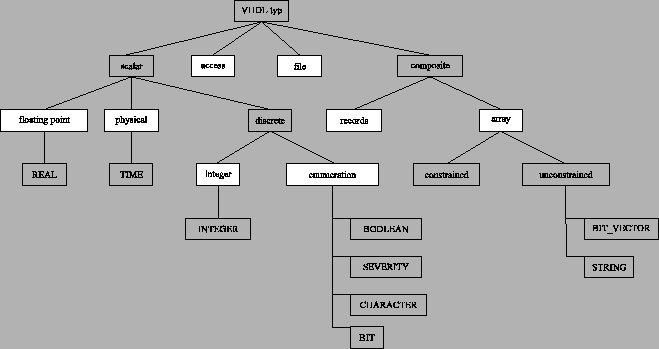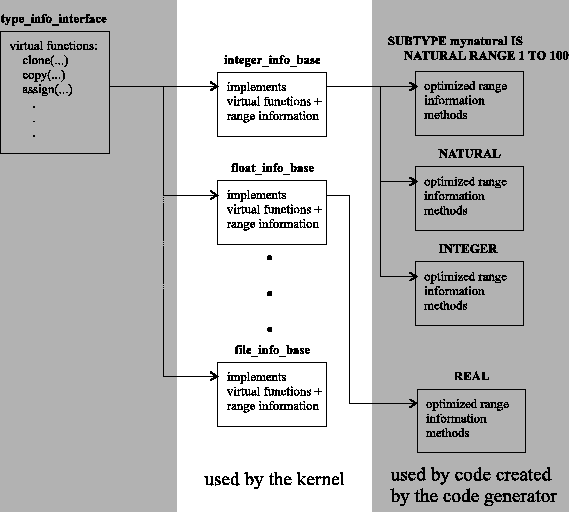Next: The Type Info Classes Up: The FreeHDL Compiler/Simulator System Previous: acl Class Instantiation

# VHDL TypesIn VHDL new data types may be defined. Each VHDL types belongs two one of the following VHDL type groups:

• integer
• enumeration
• floating point
• physical
• record
• array
• access
• file.
Figure 5.1 shows the relations between the various VHDL type groups and predefined VHDL data types. During code generation each VHDL data type is converted into a corresponding C++ class. In detail each new data type is constructed of two main components:
• A type class which stores the actual data value and implements operations on the data type (assignment, compare, ...).
• A type information class (type info class). Basically, it implements methods which are used by the simulation kernel to handle corresponding instances (compare, create, copy, remove, ...).
A type class instance is created for each corresponding VHDL object while usually there is a single type info class instance describing type properties. While the kernel uses methods provided by the type info class to handle appropriate data objects, C++ code created by the code generator exploits methods of the corresponding type class directly. Hence, the C++ compiler can perform some additional optimisations when compiling C++ code generated from VHDL source.

The simulation kernel on the other hand uses virtual functions implemented by the appropriate type information class to perform manipulation on data types. It cannot use the type class operators because they are unknown at kernel compile time.Next we discuss the structure of type info classes and type classes.

A type information class (type info class) consists of three stages (see Figure 5.2). The first base class is type_info_interface. It declares virtual functions which are used by the simulation kernel. Derived from type_info_interface a separate type info base class for each of the VHDL type groups (integer, enumeration, floating point, physical, record, array, access, and file) is defined. Finally, for each concrete VHDL type a separate type info class is derived from the corresponding type info base class. It adds some optimised methods to access type informations. Note, the methods added by this class are mainly used by code emitted by the code generator. E.g. the type info base class of an integer type includes some members to store lower and upper bounds of the type. Additionally, the corresponding type info class derived from the type info base class adds some static methods which directly return the lower and upper bounds without accessing the corresponding members of the base class. This can be done because integer bounds are locally static in VHDL, hence they can be calculated at compile time. While code emitted form the code generator uses this special methods to query type bounds the simulation kernel does not know the derived type info class. Hence, it has to read the members of the type info base class in order to get the type bounds.

For each data object a instance from a appropriate type calls is created. The type class is derived from the corresponding type base class which exists for each VHDL type group (integer, enumeration, floating point, physical, record, array, access, and file). These base classes include all data which are necessary to store the actual data value. The derived type class implements operators and methods to perform range checking and type conversion. Note, all type classes belonging to the same VHDL type group are derived from the same type base class. Hence, assignments i.e. between different VHDL integer types are allowed on C++ level even though they are prohibited by the VHDL standard. It is the task of the VHDL compiler to reject such illegal VHDL operations before code generation starts.

Actually, the derived type classes are constructed by C++ templates as shown in the example below. The parameter to the template is a type info class. The static members of the type class can now be used in the template to query type information e.g. to determine lower, upper, left, or right bound of the type. Actually, the parameter class is simply used to pass some type informations to the template.

Example:

// The type_info_interface class. All type information classes are
// derived from this base class.
class type_info_interface {
public:
virtual void *clone(const void *src); // A method to clone a data
// instance
virtual void *copy(void *dest, const void *src);  // A method to
// copy data
...
};

// A type info base class for integer types. Note, for each base VHDL
// type group a separate info base class exists.
class integer_info_base : public type_info_interface {
public:
// Some class members to store type bounds
int left_bound, right_bound, low_bound, high_bound;

void *clone(const void *src) { ... }; // Implementation of clone for
// integer types
void *copy(void *dest, const void *src) { ... } // Implementation of
// copy for integer types
...
};

// The type info class for the predefined VHDL integer type NATURAL''.
class L3std_Q8standard_I7natural : public integer_info_base {
public:
// Some optimised methods to get the bounds of natural''. Note,
// type bounds are locally static, hence the VHDL compiler can
// determine them at compile time. Actually, e.g. low() returns the
// same value as stored in low_bound. However, executing low()
// can be inlined and is then faster than reading the corresponding
// member low_bound of the base class.
static int low() { return 0; }
static int high() { return 2147483647; }
static int left() { return 0; }
static int right() { return 2147483647; }
L3lib_Q8standard_I7natural() :
integer_info_base(left(), right(), low(), high()) {};
}

};

// Base class for all integer types
class integer_base {
public:
int value;
integer_base(int a) { value = a; };
integer_base(const integer_base &a) { value = a.value; };
integer_base &operator=(integer_base a) {
value = a.value;
return *this;
}
static int ld_size() { return INTEGER_LD_SIZE; }
static bool scalar() { return true; }
static void cleanup() {}; /* Dummy function */
}

};

// Template to create an integer type. Note, R represents a type
// info class.
template<class R> class integer_type : public integer_base {
public:
// Note, R::left() is static and returns a constant, hence the
// compiler can directly replace the function call by the
// corresponding constant value (see class
// L3std_Q8standard_I7natural).
integer_type(const int a=R::left()) : integer_base(a) {...};
integer_type &operator=(const integer_type a);
integer_type(const integer_base a) : integer_base(a) {};
integer_type &init(const type_info_interface *tinfo, void *p = NULL) {...}

/** Predefined VHDL integer operators */
/* Arithmetical operators */
integer_type operator+(const integer_type a) {
// Note,
if ((long)value + (long)a.value > (long)R::high())
error(ERROR_INTEGER_OVERFLOW);
return integer_type(value + a.value)
}
integer_type operator-(const integer_type a) {...};
integer_type operator*(const integer_type a) {...};
integer_type operator/(const integer_type a) {...};
/* Compare operators */
int operator<(const integer_type a) {...};
int operator<=(const integer_type a) {...};
int operator>(const integer_type a) {...};
int operator>=(const integer_type a) {...};
int operator==(const integer_type a) {...};
int operator!=(const integer_type a) {...};
};

// To enhance readability of automatically generated code,
// a define statement maps the natural'' type name to the
// corresponding template instantiation
#define L3std_Q8standard_T7natural   integer_type<L3std_Q8standard_I7natural>

// Some variables of natural'' type
L3std_Q8standard_T7natural obj1;
L3std_Q8standard_T7natural obj2(2);
L3std_Q8standard_T7natural obj3(obj1);Next: The Type Info Classes Up: The FreeHDL Compiler/Simulator System Previous: acl Class Instantiation

1998-11-17